Home > CC2MN > Chapter cc27 > Lesson cc27.1.6 > Problem7-71

7-71.
1. Find the value of x that makes each equation true. Homework Help ✎

1. –4.9x = 9.8

2.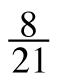= −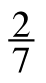x

3.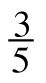+ x =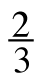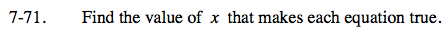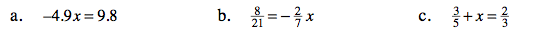To solve for x, divide both sides by −4.9.

$\frac{-4.9\textit{x}}{-4.9}=\frac{9.8}{-4.9}$

x = −2

$\text{Multiply both sides by }-\frac{7}{2}.$

$\textit{x}=-\frac{4}{3}$

You will need to subtract three fifths from both sides to isolate x.